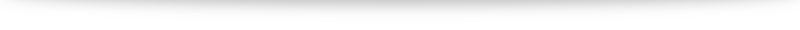# Proof of the dot product formula

## My experiences with the dot product

I struggled quite a bit when I first encountered the dot/scalar product in school. The dot product between two vectors $\mathbf{a}$ and $\mathbf{b}$ was defined to be $\mathbf{a} \cdot \mathbf{b} = |\mathbf{a}||\mathbf{b}| \cos \theta$ where $\theta$ is the angle between the two vectors. This definition posed a bit of stumbling block for me. Up until this point, most definitions has come to be pretty intuitive (and if not at first, it starts feeling reasonably natural usually within a week or two of working with it). This formula or definition seems like it is simply plucked out of midair.